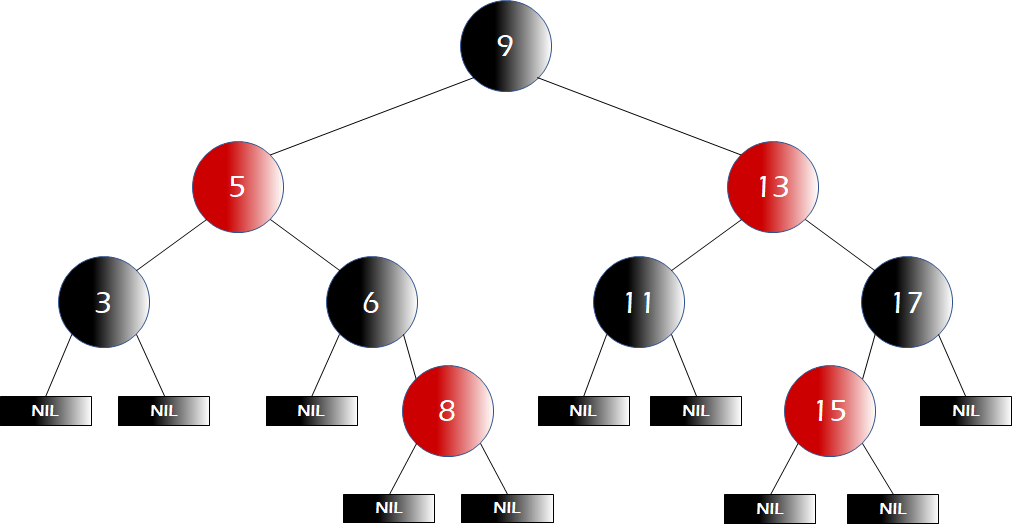(Public / Wikimedia Commons) Trabuco Hills High School

# Opinion: std::map vs std::unordered_map

Computer programs are often used to store and access large amounts of data, with one example being a program to manage students’ data. If a teacher wanted to modify a student’s information, they would want to find the student quickly and update it. They’d also want to pull up a list of their students for attendance, sorted by student ID. There are two great containers in the C++ standard for these use cases: the map and the unordered map.

The unordered map (std::unordered_map to be precise) would be better for the first use case in the above example. This is a container class that “contains key-value pairs with unique keys.” The unique keys are used to access the paired values, which is how the container finds the student. It is usually implemented using the hash table.(John Song)

This method uses an array of buckets to store data. The index is computed using the hash function that converts a key into a hash, and the data is stored in a bucket associated with the index. In case of a collision where the hash function returns the same value for multiple keys, the table stores a list for each hash value to accommodate multiple keys with the same hash value. Thus, searches are typically very fast (at a constant time – O(1) in Big O notation), although the worst-case scenario is when all values end up with the same hash value, requiring a linear search through the whole set.

The std::map, a.k.a. “ordered map” or just “map,” is more fit for the attendance list case above. The map is also a data structure meant for storing data with a key-value mapping mechanism, but unlike the std::unordered_map, the std::map is usually implemented using red-black trees, according to C++ Standard Reference (cppreference.com). Red-black trees originated from binary search trees where you can easily retrieve the data in order, according to “Introduction to Algorithms.”(John Song)

A red-black tree is a type of balanced binary search tree. A binary search tree is made up of nodes, starting from the root node. Each node has two child nodes, left and right, according to “Introduction to Algorithms.” The left child node is lesser in value than the parent, while the right child is greater, resulting in a sorted data set at any given time (this will change depending on whether you want an ascending order or a descending order), according to “Introduction to Algorithms.”

The search operation does not run at a constant time — the number of comparisons needed in the worst case can be asymptotically expressed as log n, with n being the number of elements – O(log n). For example, if there was a perfectly balanced tree with 7 elements, or 23 – 1, the comparisons required to reach a leaf node is 3, and if we had another tree with 15 elements, 24 – 1 in this case, the comparisons needed is 4 to reach a bottom element (yes, we’re talking about the worst case). As you can see, the number of comparisons needed increases logarithmically.

Hashing is faster than trees when searching; you’ll want to use it in cases where you don’t need to keep the order but you just want to quickly find data. Trees are a better bet when you want to have all your data always ordered so that you can easily get sorted lists on demand. It is important to know the pros and cons of each data structure so you can choose the right one in different situations, if you wouldn’t like to make the same mistake I did.